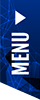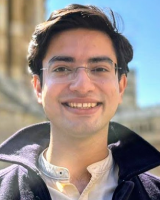# On the Expressive Power of Geometric Graph Neural Networks

[CFAR Outstanding PhD Student Seminar Series]
On the Expressive Power of Geometric Graph Neural Networks by Chaitanya K. Joshi
15 Feb 2023 | 4.00pm (Singapore Time)

The expressive power of Graph Neural Networks (GNNs) has been studied extensively through the Weisfeiler-Leman (WL) graph isomorphism test. However, standard GNNs and the WL framework are inapplicable for geometric graphs embedded in Euclidean space, such as biomolecules, materials, and other physical systems.

In this seminar, Chaitanya K. Joshi will share a recent paper titled “On the Expressive Power of Geometric Graph Neural Networks” where he and the team proposed a geometric version of the WL test (GWL) for discriminating geometric graphs while respecting the underlying physical symmetries: permutations, rotation, reflection, and translation. They used GWL to characterise the expressive power of geometric GNNs that are invariant or equivariant to physical symmetries in terms of distinguishing geometric graphs. He will conclude the talk by explaining how GWL unpacks the ways key design choices influence geometric GNN expressivity: (1) Invariant layers have limited expressivity as they cannot distinguish one-hop identical geometric graphs; (2) Equivariant layers distinguish a larger class of graphs by propagating geometric information beyond local neighbourhoods; (3) Higher order tensors and scalarisation enable maximally powerful geometric GNNs; and (4) GWL’s discrimination-based perspective is equivalent to universal approximation. Synthetic experiments supplementing their results are available at https://github.com/chaitjo/geometric-gnn-dojo.